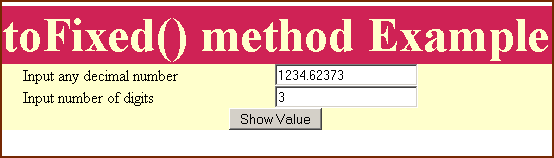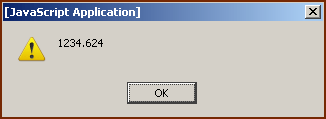Tutorials

# JavaScript toFixed method

In JavaScript, toFixed() method returns a string representation of number according to the post decimal digits. It will fix the number of precision digits after the decimal point according to the passed digits in arguments.

Syntax :

 numberObject.toFixed(digitsAfterDecimalPosition);

Where numberObject is the number at which we will apply toFixed() method and digitsAfterDecimalPosition are the number of post decimal digits for precision. If no argument is passed to this method it assumes it to be "0".

Explanation :

To illustrate toFixed() method we have created a simple HTML page which has two input text boxes and these text boxes are taking number value and post decimal digits. Suppose we have applied toFixed() method over a digit "1234.567" and post decimal digit is "2". It will result as "1234.57" after rounding the third post decimal digit.

Code :

In HTML page we have a button "Show Value" and it calls the function "callToFixed()" on the click event of button.

 ``` function callToFixed(){ var val= new Number(document.getElementById("txt").value); var digitsToFixed= document.getElementById("txtfxd").value; alert(val.toFixed(digitsToFixed)); } ```

In the first line of code we have taken the number into a variable "val" and second line of the function takes the number of digits for the post decimal precision digits into the variable "digitsToFixed". Now since we have both values we can apply toFixed() method over it.

 ``` toFixed() method Example
toFixed() method Example
Input any decimal number
Input number of digits
```

Output :Input any decimal number and digits for precision and click on the "Show Value" button.Suppose we have entered decimal number 1234.62373 and post decimal digit is 3 and after calling toFixed() method result will 1234.624 i.e. the third digit is the result of rounding the fourth digit.And if we insert a decimal number which has the number of post decimal digits less than the inserted post decimal digits then it will automatically add "0" to the last of the decimal numbers as you can see from the output.